Updating search results...

# 12 Results

View
Selected filters:
• formulasConditional Remix & Share Permitted
CC BY-NC
Rating
0.0 stars
Rating
0.0 stars
Subject:
Mathematics
Material Type:
Full Course
Provider:
Pearson
11/02/2020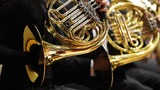Conditional Remix & Share Permitted
CC BY-NC
Rating
0.0 stars

Rate

Type of Unit: Concept

Prior Knowledge

Students should be able to:

Solve problems involving all four operations with rational numbers.
Understand quantity as a number used with a unit of measurement.
Solve problems involving quantities such as distances, intervals of time, liquid volumes, masses of objects, and money, and with the units of measurement for these quantities.
Understand that a ratio is a comparison of two quantities.
Write ratios for problem situations.
Make and interpret tables, graphs, and diagrams.
Write and solve equations to represent problem situations.

Lesson Flow

In this unit, students will explore the concept of rate in a variety of contexts: beats per minute, unit prices, fuel efficiency of a car, population density, speed, and conversion factors. Students will write and refine their own definition for rate and then use it to recognize rates in different situations. Students will learn that every rate is paired with an inverse rate that is a measure of the same relationship. Students will figure out the logic of how units are used with rates. Then students will represent quantitative relationships involving rates, using tables, graphs, double number lines, and formulas, and they will see how to create one such representation when given another.

Subject:
Algebra
Mathematics
Provider:
Pearson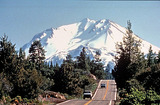Conditional Remix & Share Permitted
CC BY-NC
Rating
0.0 stars

In this lesson, students represent quantitative relationships involving rates using tables, graphs, double number lines, and formulas. Students will understand how to create one such representation when given another representation.Key ConceptsQuantitative relationships involving rates can be represented using tables, graphs, double number lines, and formulas. One such representation can be used to create another representation. Two rates can describe each situation: the rate and its inverse. For the water pump situation, there are two related formulas: a formula for finding the quantity of water pumped for any amount of time, and a formula for finding the amount of time for any quantity of water.Goals and Learning ObjectivesUnderstand that tables, graphs, double number lines, and formulas can be used to represent the same situation.Compare the different representations within a situation and the same representation across similar situations.Understand each representation and how to find the rate in each one.

Subject:
Algebra
Material Type:
Lesson Plan
Author:
Pearson
11/02/2020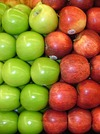Conditional Remix & Share Permitted
CC BY-NC
Rating
0.0 stars

In this lesson, students use their knowledge of rates, graphs of rates, and formulas to solve problems.Key ConceptsThe formula for a rate is a mathematical way of writing a rule for computing a value. Rate formulas describe a constant relationship between two quantities. Each point on the graph of a rate shows a pair of related values. A graph of a constant rate is a straight line.Goals for Learning ObjectivesUncover any partial understandings and misconceptions students have about rate, graphs of rates, and formulas.Develop a more robust understanding of rate.Help identify which Gallery problems students should work on.

Subject:
Algebra
Material Type:
Lesson Plan
Author:
Pearson
11/02/2020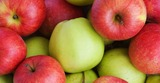Conditional Remix & Share Permitted
CC BY-NC
Rating
0.0 stars

In this lesson, students write formulas to represent different rate relationships.Key ConceptsA formula is a mathematical way of writing a rule for computing a value.Formulas, like c = 2.50w or d = 20g, describe the relationship between quantities.The formula c = 2.50w describes the relationship between a cost and a quantity that costs \$2.50 per unit of weight. Here, w stands for any weight, and c stands for the cost of w pounds at \$2.50 per pound.The formula d = 20g describes the relationship between the distance, d, and the number of gallons of gas, g, for a car that gets 20 miles per gallon.Goals and Learning ObjectivesUse equations with two variables to express relationships between quantities that vary together.

Subject:
Algebra
Material Type:
Lesson Plan
Author:
Pearson
11/02/2020Conditional Remix & Share Permitted
CC BY-NC
Rating
0.0 stars

Surface Area and Volume

Type of Unit: Conceptual

Prior Knowledge

Students should be able to:

Identify rectangles, parallelograms, trapezoids, and triangles and their bases and heights.
Identify cubes, rectangular prisms, and pyramids and their faces, edges, and vertices.
Understand that area of a 2-D figure is a measure of the figure's surface and that it is measured in square units.
Understand volume of a 3-D figure is a measure of the space the figure occupies and is measured in cubic units.

Lesson Flow

The unit begins with an exploratory lesson about the volumes of containers. Then in Lessons 2–5, students investigate areas of 2-D figures. To find the area of a parallelogram, students consider how it can be rearranged to form a rectangle. To find the area of a trapezoid, students think about how two copies of the trapezoid can be put together to form a parallelogram. To find the area of a triangle, students consider how two copies of the triangle can be put together to form a parallelogram. By sketching and analyzing several parallelograms, trapezoids, and triangles, students develop area formulas for these figures. Students then find areas of composite figures by decomposing them into familiar figures. In the last lesson on area, students estimate the area of an irregular figure by overlaying it with a grid. In Lesson 6, the focus shifts to 3-D figures. Students build rectangular prisms from unit cubes and develop a formula for finding the volume of any rectangular prism. In Lesson 7, students analyze and create nets for prisms. In Lesson 8, students compare a cube to a square pyramid with the same base and height as the cube. They consider the number of faces, edges, and vertices, as well as the surface area and volume. In Lesson 9, students use their knowledge of volume, area, and linear measurements to solve a packing problem.

Subject:
Geometry
Mathematics
Provider:
Pearson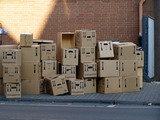Conditional Remix & Share Permitted
CC BY-NC
Rating
0.0 stars

Lesson OverviewStudents build prisms with fractional side lengths by using unit-fraction cubes (i.e., cubes with side lengths that are unit fractions, such as 13 unit or 14 unit). Students verify that the volume formula for rectangular prisms, V = lwh or V = bh, applies to prisms with side lengths that are not whole numbers.Key ConceptsIn fifth grade, students found volumes of prisms with whole-number dimensions by finding the number of unit cubes that fit inside the prisms. They found that the total number of unit cubes required is the number of unit cubes in one layer (which is the same as the area of the base) times the number of layers (which is the same as the height). This idea was generalized as V = lwh, where l, w, and h are the length, width, and height of the prism, or as V = Bh, where B is the area of the base of the prism and h is the height.Unit cubes in each layer = 3 × 4Number of layers = 5Total number of unit cubes = 3 × 4 × 5 = 60Volume = 60 cubic unitsIn this lesson, students extend this idea to prisms with fractional side lengths. They build prisms using unit-fraction cubes. The volume is the number of unit-fraction cubes in the prism times the volume of each unit-fraction cube. Students show that this result is the same as the volume found by using the formula.For example, you can build a 45-unit by 35-unit by 25-unit prism using 15-unit cubes. This requires 4 × 3  × 2, or 24, 15-unit cubes. Each 15-unit cube has a volume of 1125 cubic unit, so the total volume is 24125 cubic units. This is the same volume obtained by using the formula V = lwh:V=lwh=45×35×25=24125.15-unit cubes in each layer = 3 × 4Number of layers = 2Total number of 15-unit cubes = 3 × 4 × 2 =  24Volume = 24 × 1125 = 24125 cubic units Goals and Learning ObjectivesVerify that the volume formula for rectangular prisms, V = lwh or V = Bh, applies to prisms with side lengths that are not whole numbers.

Subject:
Geometry
Material Type:
Lesson Plan
Author:
Pearson
11/02/2020Conditional Remix & Share Permitted
CC BY-NC
Rating
0.0 stars

Four full-year digital course, built from the ground up and fully-aligned to the Common Core State Standards, for 7th grade Mathematics. Created using research-based approaches to teaching and learning, the Open Access Common Core Course for Mathematics&nbsp;is designed with student-centered learning in mind, including activities for students to develop valuable 21st century skills and academic mindset.

Subject:
Mathematics
Material Type:
Full Course
Provider:
Pearson
11/02/2020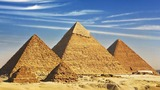Conditional Remix & Share Permitted
CC BY-NC
Rating
0.0 stars

Zooming In On Figures

Unit Overview

Type of Unit: Concept; Project

Length of Unit: 18 days and 5 days for project

Prior Knowledge

Students should be able to:

Find the area of triangles and special quadrilaterals.
Use nets composed of triangles and rectangles in order to find the surface area of solids.
Find the volume of right rectangular prisms.
Solve proportions.

Lesson Flow

After an initial exploratory lesson that gets students thinking in general about geometry and its application in real-world contexts, the unit is divided into two concept development sections: the first focuses on two-dimensional (2-D) figures and measures, and the second looks at three-dimensional (3-D) figures and measures.
The first set of conceptual lessons looks at 2-D figures and area and length calculations. Students explore finding the area of polygons by deconstructing them into known figures. This exploration will lead to looking at regular polygons and deriving a general formula. The general formula for polygons leads to the formula for the area of a circle. Students will also investigate the ratio of circumference to diameter ( pi ). All of this will be applied toward looking at scale and the way that length and area are affected. All the lessons noted above will feature examples of real-world contexts.
The second set of conceptual development lessons focuses on 3-D figures and surface area and volume calculations. Students will revisit nets to arrive at a general formula for finding the surface area of any right prism. Students will extend their knowledge of area of polygons to surface area calculations as well as a general formula for the volume of any right prism. Students will explore the 3-D surface that results from a plane slicing through a rectangular prism or pyramid. Students will also explore 3-D figures composed of cubes, finding the surface area and volume by looking at 3-D views.
The unit ends with a unit examination and project presentations.

Subject:
Geometry
Mathematics
Provider:
Pearson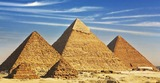Conditional Remix & Share Permitted
CC BY-NC
Rating
0.0 stars

Students discover the formula for finding the volume of a pyramid and apply the formula to solve problems.Key ConceptsThe volume of a pyramid is one-third the volume of a prism with the same base and height. The shape of the base does not matter (including if it’s a circle), and students will see the same one-third comparison between a cylinder and cone.GoalsUnderstand the formula for the volume of a pyramid.Apply the volume formula to solve problems.

Subject:
Geometry
Material Type:
Lesson Plan
Author:
Pearson
11/02/2020Conditional Remix & Share Permitted
CC BY-NC
Rating
0.0 stars

Lesson OverviewStudents will compare the formula for the area of a regular polygon to discover the formula for the area of a circle.Key ConceptsThe area of a regular polygon can be found by multiplying the apothem by half of the perimeter. If a circle is thought of as a regular polygon with many sides, the formula can be applied.For a circle, the apothem is the radius, and p is C.A=a(p2)→A=rC2→A=rπd2→A=rπ2r2→A=rπr=πr2 GoalsDerive the formula for the area of a circle.Apply the formula to find the area of circles.SWD: Consider the prerequisite skills for this lesson: understanding and applying the formula for the area of a regular polygon. Students with disabilities may need direct instruction and guided practice with this skill.Students should understand these domain-specific terms:apothemparallelogramderivationheightapproximate (estimate)scatter plotpiperimetercircumferenceIt may be helpful to preteach these terms to students with disabilities.

Subject:
Geometry
Material Type:
Lesson Plan
Author:
Pearson
11/02/2020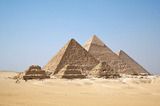Conditional Remix & Share Permitted
CC BY-NC
Rating
0.0 stars

Students will extend their knowledge of surface area and nets of rectangular prisms to generalize a formula for the surface area of any prism.Key ConceptsStudents know how to find the surface area of a rectangular prism using a net and adding the areas for pairs of congruent faces. Students have not seen that the lateral surface forms one long rectangle whose length is the perimeter of the base and whose width is the height of the prism.Using this idea, the surface area of any right prism can be found using the formula:SA = 2B + (perimeter of the base)hGoalsFind a general formula for surface area of prisms.Find the surface area of different prisms.SWD: Generalization of skills can be particularly challenging for some students with disabilities. Students may need direct instruction on the connection between what they already understand and a general formula.Some students with disabilities may have difficulty recalling formulas when it comes time to apply them. Once students discover the formula SA = 2B + (perimeter of the base)h, consider posting the formula in the classroom and encouraging students to add the formula(s) to the resources they have available when completing classwork and homework.

Subject:
Geometry
Material Type:
Lesson Plan
Author:
Pearson